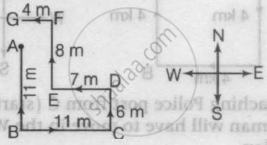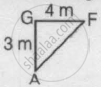# How Far is Point F from Point A? - Logical Reasoning

MCQ

Study the given information and answer the given question.

• Point A is 11m North of point B.
• Point C is 11m East of point B.
• Point D is 6m North of point C.
• Point E is 7m West of point D.
• Point F is 8m North of point E.
• Point G is 4m West of point F.

How far is point F from point A?

• 43m

• 4m

• 3m

• 5m

#### Solution

5m
Explanation:According to the question,AG = BG - AB = (CD + EF) - (AB)
= (6 + 8) - 11 = 3m and GF = 4m
∴ AF = sqrt(AG^2+GF^2)=sqrt(9+16)=5m

Concept: Direction Sense Test
Is there an error in this question or solution?